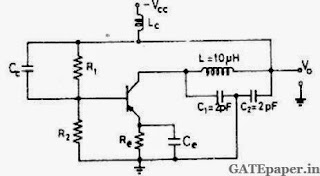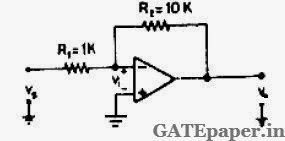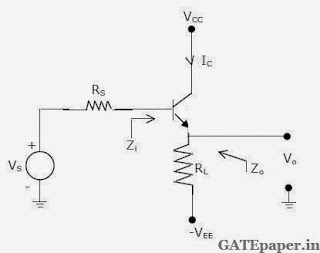### GATE 2001 ECE Video Solutions on Analog Circuits (Analog Electronics)

1. The current gain of a BJT is
a. gmro
b. gm / ro
c. gmrπ
d. gm / rπ

2. The ideal OP-AMP has the following characteristics
a. Ri = ∞, AV= ∞, Ro = 0
b. Ri = 0, AV= ∞, Ro = 0
c. Ri = ∞, AV= ∞, Ro = ∞
d. Ri = 0, AV= ∞, Ro = ∞

3. Consider the following two statements :
Statement 1: Astable Multivibrator can be used for generating square wave.
Statement 2: Bistable Multivibrator can be used for storing binary information.
a. Only statement 1 is correct.
b. Only statement 2 is correct.
c. Both the statements 1 and 2 are correct.
d. Both the statements 1 and 2 are incorrect.

4. An NPN BJT has gm = 38 mA/volt, Cµ = 10-14 F, Cπ = 4x10-13 F and DC current gain β = 90. For this transistor fτ and fβ are
a. fτ = 1.64 x 108 Hz and fβ = 1.47 x 1010 Hz
b. fτ = 1.47 x 1010 Hz and fβ = 1.64 x 108 Hz
c. fτ = 1.33 x 1012 Hz and fβ = 1.47 x 1010 Hz
d. fτ = 1.47 x 1010 Hz and fβ = 1.33 x 1012 Hz

5. The following circuit shown in the figure isa. Hartley oscillator with fosc = 79.6MHz
b. Colpitts oscillator with fosc = 50.3MHz
c. Hartley oscillator with fosc = 159.2MHz
d. Colpitts oscillator with fosc = 159.2MHz

6. The inverting OP-AMP shown in the figure has an open loop gain of 100. The closed loop gain Vo/Vsisa. – 8
b. – 9
c. – 10
d. – 11

7. In the figure, assume the OP-AMPs to be ideal. The output Vo of the circuit is8. An emitter follower amplifier is shown below, where Zi is the impedance looking into the base of the transistor and Zo is the impedance looking into the emitter of the transistor.a. Draw the small signal equivalent circuit of the amplifier.
b. Obtain an expression for Zi.
c. Obtain an expression for Zo.
d. Determine Zi and Zo, if a capacitor C is connected across R1.

9. In the circuit shown, assume that the OP-AMP is ideal.a. Obtain an expression for Vo in terms of Vs, R and the reverse saturation current Is of the transistor.
b. If R = 1 Ω, Is = 1 pA and the thermal voltage VT = 25 mV, then what is the value of the output voltage Vo for an input voltage Vs of 1 volt?
c. Suppose that the transistor in the feedback path is replaced by a PN junction diode with a reverse saturation current of Is. The P-side of the diode is connected to node A and the N-side to node B. then what is the expression for Vo in terms of Vs, R and Is ?